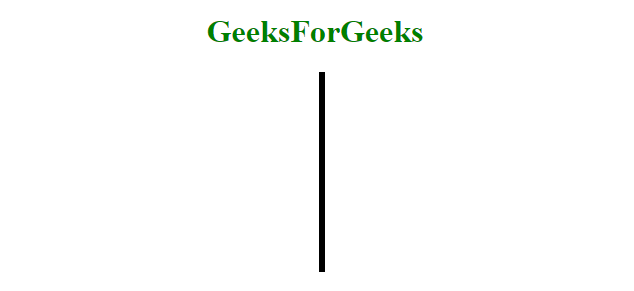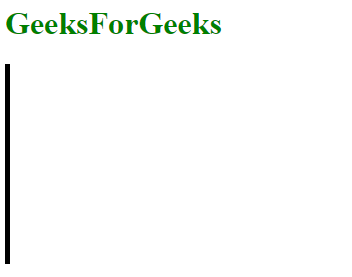# How to make a vertical line using HTML ?

• Difficulty Level : Medium
• Last Updated : 10 May, 2022

To make a vertical line, use border-left or border-right property. The height property is used to set the height of border (vertical line) element. Position property is used to set the position of vertical line.

Example 1: It creates a vertical line using border-left, height and position property.

## html

 ` ` `<``html``> `   `<``head``> ` `    ``<``title``> ` `        ``HTML border Property` `    `` ` `    `  `    ``` `    ``<``style``>` `        ``.vertical {` `            ``border-left: 6px solid black;` `            ``height: 200px;` `            ``position:absolute;` `            ``left: 50%;` `        ``}` `    ``` ``   `<``body` `style` `= ``"text-align: center;"``> `   `    ``<``h1` `style` `= ``"color: green;"``> ` `        ``GeeksForGeeks ` `    `` ` `        `  `    ``<``div` `class` `= ``"vertical"``>`   ` `   `                                      `

Output:Example 2: It creates a vertical line using border-left and height property.

## html

 ` ` `<``html``> `   `<``head``> ` `    ``<``title``> ` `        ``HTML border Property` `    `` ` `    `  `    ``` `    ``<``style``>` `        ``.vertical {` `            ``border-left: 5px solid black;` `            ``height: 200px;` `        ``}` `    ``` ``   `<``body``> `   `    ``<``h1` `style``= ``"color: green;"``> ` `        ``GeeksForGeeks ` `    `` ` `        `  `    ``<``div` `class``= ``"vertical"``>`   ` `   `                    `

Output:Supported Browsers: